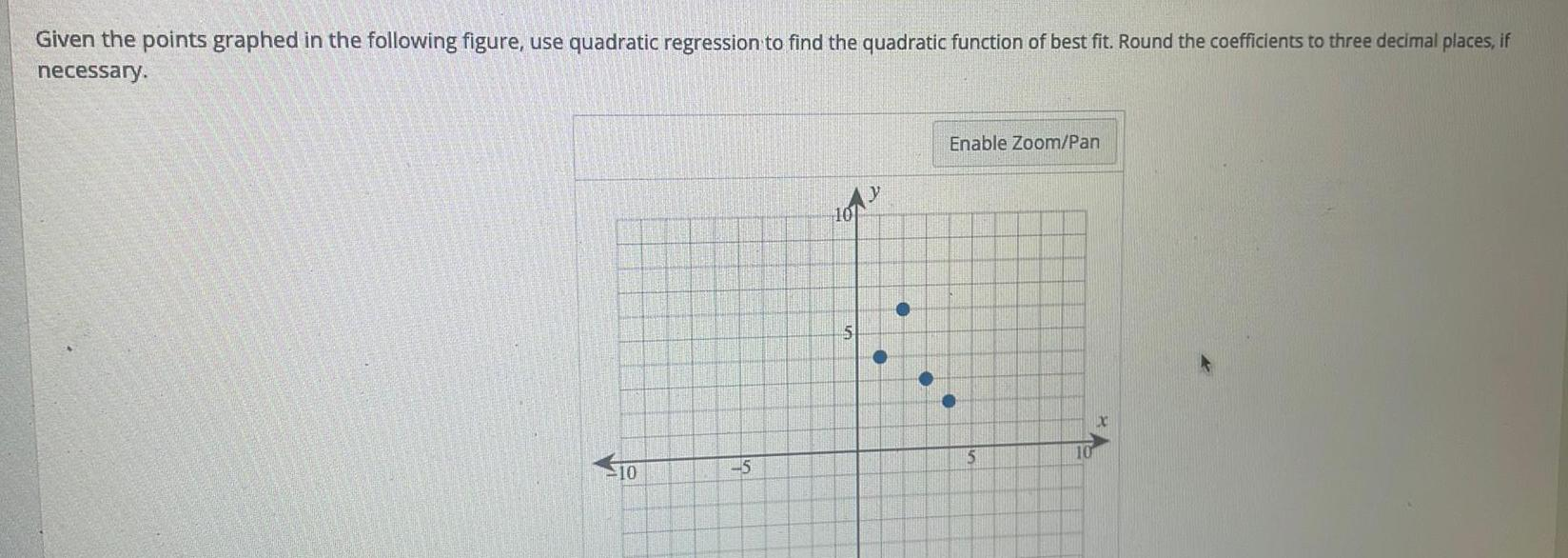Question:

# Given the points graphed in the following figure use

Last updated: 9/17/2023Given the points graphed in the following figure use quadratic regression to find the quadratic function of best fit Round the coefficients to three decimal places if necessary 10 5 5 y Enable Zoom Pan 5 10 x